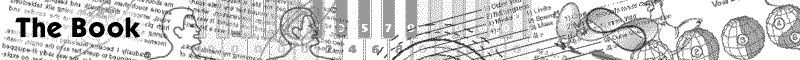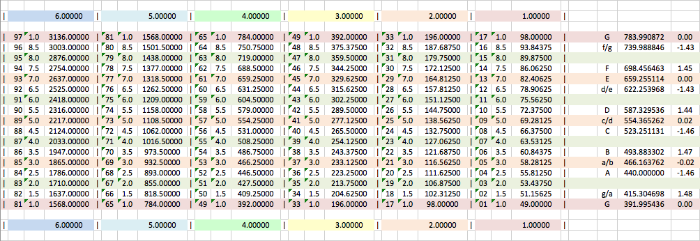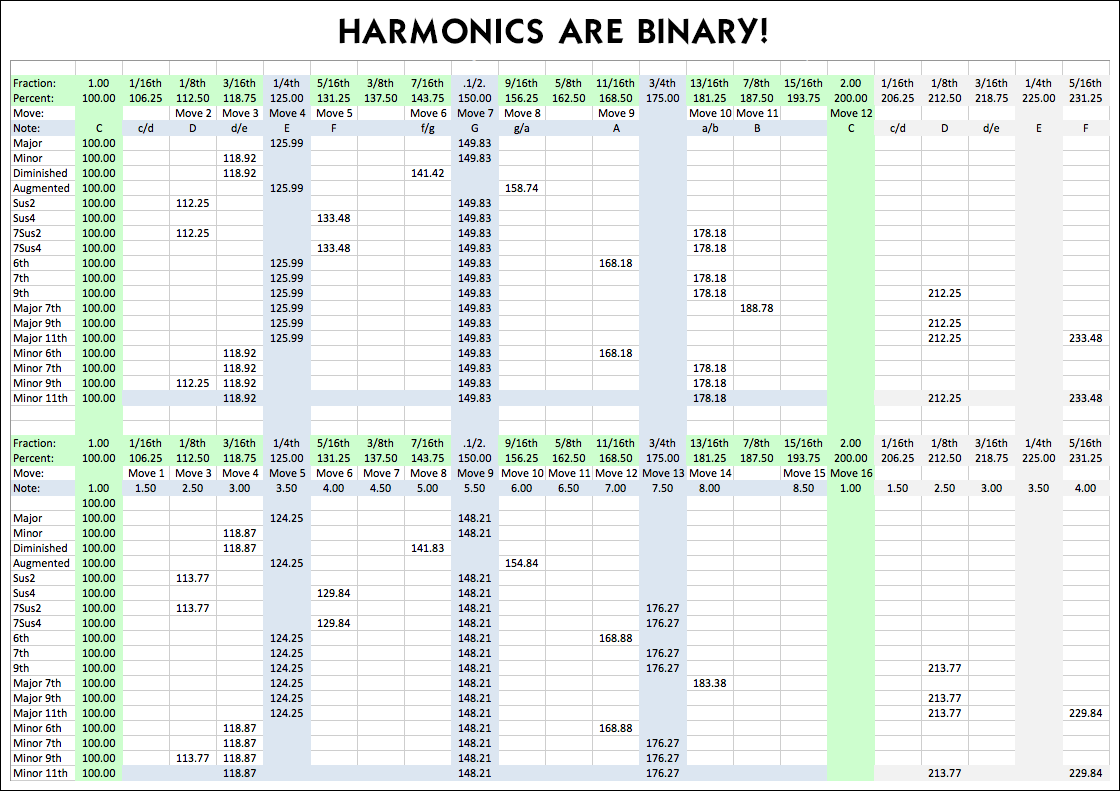V

# NOTE: SINCE WRITING ALL THIS AND PUBLISHING IT IN MY BOOK, I HAVE DONE SOME RETHINKING AND REWORKING OF THE FREQUENCIES. THESE NOTES ARE NEW. THEY ARE ROUNDED OFF FROM EQUAL TEMPERMENT - STILL CLOSE TO WHAT WAS PREVIOUSLY PUBLISHED, BUT WITH FRACTIONS THAT RESOLVE TO .5, .25, .125, .0625 ETC. SO THAT HARMONIES ARE MORE PRECISE.# Here are some suggestions for harmonies: Use the bottom portion of this chart:# For those wanting to know what note each of these notes is close to in traditional music, the right hand column of each table has indicators that are rough approximations.

Melodies Harmonies
......v ......v
 97 = 1 3136.0 G 96 = -- 3003.0 f/g 95 = 8 2876.0 New 94 = -- 2754.0 F 93 = 7 2637.0 E 92 = -- 2525.0 d/e 91 = 6 2418.0 New 90 = -- 2316.0 D 89 = 5 2217.0 c/d 88 = -- 2124.0 C 87 = 4 2033.0 New 86 = -- 1947.0 B 85 = 3 1865.0 a/b 84 = -- 1786.0 A 83 = 2 1710.0 New 82 = -- 1637.0 g/a 81 = 1 G 80 = -- f/g 79 = 8 New 78 = -- F 77 = 7 E 76 = -- d/e 75 = 6 New 74 = -- D 73 = 5 c/d 72 = -- C 71 = 4 New 70 = -- B 69 = 3 a/b 68 = -- A 67 = 2 New 66 = -- g/a 65 = 1 G 64 = -- f/g 63 = 8 New 62 = -- F 61 = 7 E 60 = -- d/e 59 = 6 New 58 = -- D 57 = 5 c/d 56 = -- C 55 = 4 New 54 = -- B 53 = 3 a/b 52 = -- A 51 = 2 New 50 = -- g/a 49 = 1 G 48 = -- f/g 47 = 8 New 46 = -- F 45 = 7 E 44 = -- d/e 43 = 6 New 42 = -- D 41 = 5 c/d 40 = -- C 39 = 4 New 38 = -- B 37 = 3 a/b 36 = -- A 35 = 2 New 34 = -- g/a 33 = 1 G 32 = -- f/g 31 = 8 New 30 = -- F 29 = 7 E 28 = -- d/e 27 = 6 New 26 = -- D 25 = 5 c/d 24 = -- C 23 = 4 New 22 = -- B 21 = 3 a/b 20 = -- A 19 = 2 New 18 = -- g/a 17 = 1 G 16 = -- 93.84375 f/g 15 = 8 89.875 New 14 = -- 86.0625 F 13 = 7 82.40625 E 12 = -- 78.90625 d/e 11 = 6 75.5625 New 10 = -- 72.375 D 09 = 5 69.28125 c/d 08 = -- 66.375 C 07 = 4 63.53125 New 06 = -- 60.84375 B 05 = 3 58.2815 a/b 04 = -- 55.8125 A 03 = 2 53.4375 New 02 = -- 51.15625 g/a 01 = 1 49.00 G
 97 = 1 3136.0 G 96 = -- 3003.0 f/g 95 = 8 2876.0 New 94 = -- 2754.0 F 93 = 7 2637.0 E 92 = -- 2525.0 d/e 91 = 6 2418.0 New 90 = -- 2316.0 D 89 = 5 2217.0 c/d 88 = -- 2124.0 C 87 = 4 2033.0 New 86 = -- 1947.0 B 85 = 3 1865.0 a/b 84 = -- 1786.0 A 83 = 2 1710.0 New 82 = -- 1637.0 g/a 81 = 1 G 80 = -- f/g 79 = 8 New 78 = -- F 77 = 7 E 76 = -- d/e 75 = 6 New 74 = -- D 73 = 5 c/d 72 = -- C 71 = 4 New 70 = -- B 69 = 3 a/b 68 = -- A 67 = 2 New 66 = -- g/a 65 = 1 G 64 = -- f/g 63 = 8 New 62 = -- F 61 = 7 E 60 = -- d/e 59 = 6 New 58 = -- D 57 = 5 c/d 56 = -- C 55 = 4 New 54 = -- B 53 = 3 a/b 52 = -- A 51 = 2 New 50 = -- g/a 49 = 1 G 48 = -- f/g 47 = 8 New 46 = -- F 45 = 7 E 44 = -- d/e 43 = 6 New 42 = -- D 41 = 5 c/d 40 = -- C 39 = 4 New 38 = -- B 37 = 3 a/b 36 = -- A 35 = 2 New 34 = -- g/a 33 = 1 G 32 = -- f/g 31 = 8 New 30 = -- F 29 = 7 E 28 = -- d/e 27 = 6 New 26 = -- D 25 = 5 c/d 24 = -- C 23 = 4 New 22 = -- B 21 = 3 a/b 20 = -- A 19 = 2 New 18 = -- g/a 17 = 1 G 16 = -- 93.84375 f/g 15 = 8 89.875 New 14 = -- 86.0625 F 13 = 7 82.40625 E 12 = -- 78.90625 d/e 11 = 6 75.5625 New 10 = -- 72.375 D 09 = 5 69.28125 c/d 08 = -- 66.375 C 07 = 4 63.53125 New 06 = -- 60.84375 B 05 = 3 58.2815 a/b 04 = -- 55.8125 A 03 = 2 53.4375 New 02 = -- 51.15625 g/a 01 = 1 49.00 G